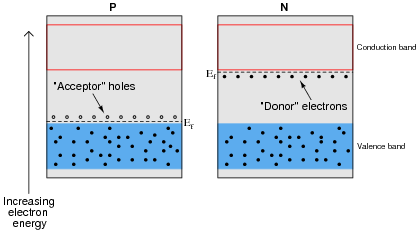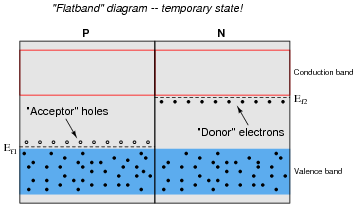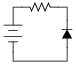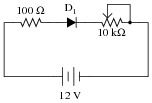# PN Junctions

## Discrete Semiconductor Devices and Circuits

• #### Question 1

 Don’t just sit there! Build something!!

Learning to mathematically analyze circuits requires much study and practice. Typically, students practice by working through lots of sample problems and checking their answers against those provided by the textbook or the instructor. While this is good, there is a much better way.

You will learn much more by actually building and analyzing real circuits, letting your test equipment provide the “answers” instead of a book or another person. For successful circuit-building exercises, follow these steps:

1. Carefully measure and record all component values prior to circuit construction, choosing resistor values high enough to make damage to any active components unlikely.
2. Draw the schematic diagram for the circuit to be analyzed.
3. Carefully build this circuit on a breadboard or other convenient medium.
4. Check the accuracy of the circuit’s construction, following each wire to each connection point, and verifying these elements one-by-one on the diagram.
5. Mathematically analyze the circuit, solving for all voltage and current values.
6. Carefully measure all voltages and currents, to verify the accuracy of your analysis.
7. If there are any substantial errors (greater than a few percent), carefully check your circuit’s construction against the diagram, then carefully re-calculate the values and re-measure.

When students are first learning about semiconductor devices, and are most likely to damage them by making improper connections in their circuits, I recommend they experiment with large, high-wattage components (1N4001 rectifying diodes, TO-220 or TO-3 case power transistors, etc.), and using dry-cell battery power sources rather than a benchtop power supply. This decreases the likelihood of component damage.

As usual, avoid very high and very low resistor values, to avoid measurement errors caused by meter “loading” (on the high end) and to avoid transistor burnout (on the low end). I recommend resistors between 1 kΩ and 100 kΩ.

One way you can save time and reduce the possibility of error is to begin with a very simple circuit and incrementally add components to increase its complexity after each analysis, rather than building a whole new circuit for each practice problem. Another time-saving technique is to re-use the same components in a variety of different circuit configurations. This way, you won’t have to measure any component’s value more than once.

• #### Question 2

The relationship between voltage and current for a PN junction is described by this equation, sometimes referred to as the “diode equation,” or “Shockley’s diode equation” after its discoverer:

 $$I_D= I_S(e^{\frac{qV_D}{NkT}} -1)$$

Where,

ID = Current through the PN junction, in amps

IS = PN junction saturation current, in amps (typically 1 picoamp)

e = Euler’s number ≈ 2.718281828

q = Electron unit charge, 1.6 ×10−19 coulombs

VD = Voltage across the PN junction, in volts

N = Nonideality coefficient, or emission coefficient (typically between 1 and 2)

k = Boltzmann’s constant, 1.38 ×10−23

T = Junction temperature, degrees Kelvin

At first this equation may seem very daunting, until you realize that there are really only three variables in it: ID, VD, and T. All the other terms are constants. Since in most cases we assume temperature is fairly constant as well, we are really only dealing with two variables: diode current and diode voltage. Based on this realization, re-write the equation as a proportionality rather than an equality, showing how the two variables of diode current and voltage relate:

 ID ∝ . . .

Based on this simplified equation, what would an I/V graph for a PN junction look like? How does this graph compare against the I/V graph for a resistor?• #### Question 3

The characteristically colored glow from a gas-discharge electric light is the result of energy emitted by electrons in the gas atoms as they fall from high-level “excited” states back to their natural (“ground”) states. As a general rule of electron behavior, they must absorb energy from an external source to leap into a higher level, and they release that energy upon returning to their original level.

Given the existence of this phenomenon, what do you suspect might be occurring inside a PN junction as it conducts an electric current?

• #### Question 4

When “P” and “N” type semiconductor pieces are brought into close contact, free electrons from the “N” piece will rush over to fill holes in the “P” piece, creating a zone on both sides of the contact region devoid of charge carriers. What is this zone called, and what are its electrical characteristics?

• #### Question 5

What happens to the thickness of the depletion region in a PN junction when an external voltage is applied to it?

• #### Question 6

Shown here are two energy diagrams: one for a “P” type semiconducting material and another for an “N” type.Next is an energy diagram showing the initial state when these two pieces of semiconducting material are brought into contact with each other. This is known as a flatband diagram:The state represented by the “flatband” diagram is most definitely a temporary one. The two different Fermi levels are incompatible with one another in the absence of an external electric field.

Draw a new energy diagram representing the final energy states after the two Fermi levels have equalized.

Note: Ef represents the Fermi energy level, and not a voltage. In physics, E always stands for energy and V for electric potential (voltage).

• #### Question 7

Draw an energy diagram for a PN semiconductor junction under the influence of a reverse external voltage.

• #### Question 8

Draw an energy diagram for a PN semiconductor junction under the influence of a forward external voltage.

• #### Question 9

Draw an energy diagram for a PN semiconductor junction showing the motion of electrons and holes conducting an electric current.

• #### Question 10

Is this diode forward-biased or reverse biased?• #### Question 11

Insert a diode into this circuit schematic in the correct direction to make it forward-biased by the battery voltage:• #### Question 12

Most introductory textbooks will tell you that a silicon PN junction drops 0.7 volts when forward-biased, and a germanium PN junction drops 0.3 volts when forward biased. Design a circuit that tests the “forward voltage” (VF) of a PN-junction diode, so you may measure the voltage yourself, without the use of a special diode-testing meter.

• #### Question 13

If a semiconductor PN junction is reverse-biased, ideally no continuous current will go through it. However, in real life there will be a small amount of reverse-bias current that goes through the junction. How is this possible? What allows this reverse current to flow?

• #### Question 14

Shockley’s diode equation in standard form is quite lengthy, but it may be considerably simplified for conditions of room temperature. Note that if the temperature (T) is assumed to be room temperature (25o C), there are three constants in the equation that are the same for all PN junctions: T, k, and q.

$$I_D= I_S(e^{\frac{qV_D}{NkT}} -1)$$

The quantity $$\frac{kT}{q}$$ is known as the thermal voltage of the junction. Calculate the value of this thermal voltage, given a room temperature of 25o C. Then, substitute this quantity into the original “diode formula” so as to simplify its appearance.

• #### Question 15

A student sets up a circuit that looks like this, to gather data for characterizing a diode:Measuring diode voltage and diode current in this circuit, the student generates the following table of data:

 Vdiode Idiode 0.600 V 1.68 mA 0.625 V 2.88 mA 0.650 V 5.00 mA 0.675 V 8.68 mA 0.700 V 14.75 mA 0.725 V 27.25 mA 0.750 V 48.2 mA

This student knows that the behavior of a PN junction follows Shockley’s diode equation, and that the equation may be simplified to the following form:

 $$I_{diode} = I_S(e^{\frac{V_{diode}}{K}} -1)$$

Where,

K = a constant incorporating both the thermal voltage and the nonideality coefficient

The goal of this experiment is to calculate K and IS, so that the diode’s current may be predicted for any arbitrary value of voltage drop. However, the equation must be simplified a bit before the student can proceed.

At substantial levels of current, the exponential term is very much larger than unity $$(e^{\frac{V_{diode}}{K}}>>1)$$, so the equation may be simplified as such:

 $$I_{diode} \approx I_S(e^{\frac{V_{diode}}{K}})$$

From this equation, determine how the student would calculate K and IS from the data shown in the table. Also, explain how this student may verify the accuracy of these calculated values.

• #### Question 16

In order to simplify analysis of circuits containing PN junctions, a “standard” forward voltage drop is assumed for any conducting junction, the exact figure depending on the type of semiconductor material the junction is made of.

How much voltage is assumed to be dropped across a conducting silicon PN junction? How much voltage is assumed for a forward-biased germanium PN junction? Identify some factors that cause the real forward voltage drop of a PN junction to deviate from its “standard” figure.

• #### Question 17

A forward-biased PN semiconductor junction does not possess a “resistance” in the same manner as a resistor or a length of wire. Any attempt at applying Ohm’s Law to a diode, then, is doomed from the start.

This is not to say that we cannot assign a dynamic value of resistance to a PN junction, though. The fundamental definition of resistance comes from Ohm’s Law, and it is expressed in derivative form as such:

$$R= \frac{dV}{dI} The fundamental equation relating current and voltage together for a PN junction is Shockley’s diode equation:$$I = I_S(e^{\frac{qV}{NkT}} -1)$$At room temperature (approximately 21 degrees C, or 294 degrees K), the thermal voltage of a PN junction is about 25 millivolts. Substituting 1 for the nonideality coefficient, we may simply the diode equation as such:$$I = I_S(e^{\frac{V}{0.025}} -1) \ \ \ \ \ \ \ \ \ \ or \ \ \ \ \ \ \ \ \ \ I = I_S (e^{40V} - 1)

Differentiate this equation with respect to V, so as to determine $$\frac{dI}{dV}$$, and then reciprocate to find a mathematical definition for dynamic resistance $$(\frac{dV}{dI})$$ of a PN junction. Hints: saturation current (IS) is a very small constant for most diodes, and the final equation should express dynamic resistance in terms of thermal voltage (25 mV) and diode current (I).

• #### Question 18

Measure the forward voltage drop of a silicon rectifying diode, such as a model 1N4001. How close is the measured forward voltage drop to the “ideal” figure usually assumed for silicon PN junctions? What happens when you increase the temperature of the diode by holding on to it with your fingers? What happens when you decrease the temperature of the diode by touching an ice cube to it?